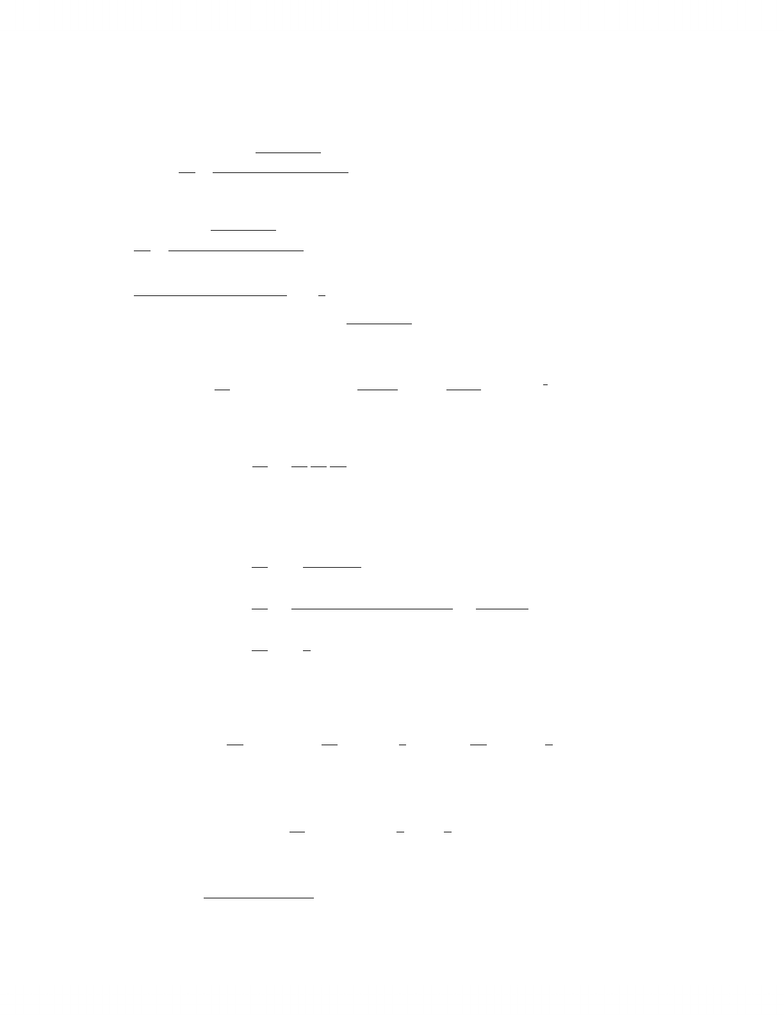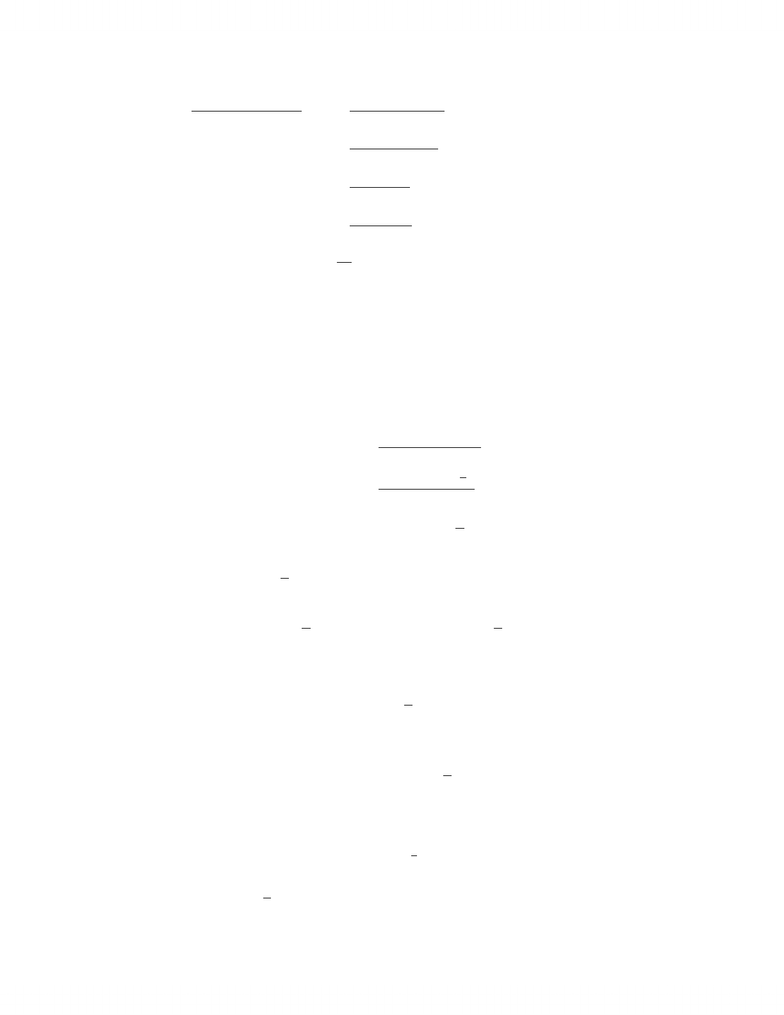# 2010 Test 2 solution

48 views7 pages
School
Department
Course
ProfessorMAT137Y, 2010-2011 Winter Session, Solutions to Term Test 2
1.Evaluate the following expressions.
(a) (7%) d
dx p1 + sec(x)
1 + cos2(x) + sin4(x)!
d
dx p1 + sec(x)
1 + cos2(x) + sin4(x)!
=1
(1 + cos2(x) + sin4(x))2×1
2(1 + sec(x))1/2sec(x) tan(x)(1 + cos2(x) + sin4(x))
p1 + sec(x)2 cos(x) sin(x) + 4 sin3(x) cos(x)
(b) (9%) Find dy
dt when t= 1 if y=1
1 + x2, x =1u
1 + u, u =t3
2
From the Chain Rule we have
dy
dt =dy
dx
dx
du
du
dt ,
and
dy
dx =2x
(1 + x2)2,
dx
du =(1)(1 + u)(1 u)(1)
(1 + u)2=2
(1 + u)2,
du
dt =3
2t5/2.
Also, when t= 1 we have u= 1 and x= 0. Therefore
dy
dxx=0 = 0,dx
duu=1 =1
2,and du
dt t=1 =3
2.
It follows that
dy
dt t=1 = (0) 1
23
2= 0.
(c) (7%) lim
x0
2x22 cos(x)
x4
1
Unlock document

This preview shows pages 1-2 of the document.
Unlock all 7 pages and 3 million more documents.lim
x0
2x22 cos(x)
x4= lim
x02x+ 2 sin(x)
4x3(by L’Hopital’s Rule)
= lim
x02 + 2 cos(x)
12x2(by L’Hopital’s Rule)
= lim
x02 sin(x)
24x(by L’Hopital’s Rule)
= lim
x02 cos(x)
24 (by L’Hopital’s Rule)
=1
12
2. (12%) Consider the function
f(x) = x+ 2x2sin(1/x),if x6= 0
0,if x= 0.
Find f0(0) if it exists, or explain why it does not exist.
Recall that f0(0), if it exists, is given by
f0(0) = lim
h0
f(0 + h)f(0)
h
= lim
h0
h+ 2h2sin 1
h
h
= lim
h01+2hsin 1
h.
Now notice that lim
h02hsin 1
h= 0. To see this, we recall that
1sin 1
h1 =⇒ −2h2hsin 1
h2h.
Since limh02h= 0 = limh02h, the Squeeze Theorem implies that
lim
h02hsin 1
h= 0.
Therefore,
f0(0) = lim
h01+2hsin 1
h= 1.
3. (12%) Water is pouring into a leaky tank at a rate of 10 m3/h. The tank is a right
circular cone with the vertex pointing down, 9 m in depth and 6 m in diameter at the top.
The water level in the tank is rising at a rate of 1
5m/h when the depth of the water is 6 m.
How fast is the water leaking out at that time?
Note: Volume of cone = 1
3πr2h, where h= height of the cone, and r= radius of the cone.
2
Unlock document

This preview shows pages 1-2 of the document.
Unlock all 7 pages and 3 million more documents.